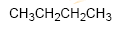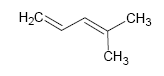Each ring or cycle of a molecule implies the loss of two hydrogens with respect to an alkane of formula CnH2n+2 . The degree of unsaturation is the number of cycles and double bonds present in a molecule.

Because alkanes contain the largest possible number of carbon-hydrogen bonds, they are said to be saturated hydrocarbons. Alkenes and cyclic compounds are unsaturated hydrocarbons.The total number of π bonds and cycles in a molecule is called the degree of unsaturation .Degree of unsaturation in compounds containing halogens, oxygens and nitrogens:

Halogens: Add the number of halogens to the number of hydrogens.
Oxygens: Ignore the oxygens.
Nitrogens : Subtract the number of nitrogens from the number of hydrogens.

Example 1: Calculate the degree of unsaturation in the following formulas :

a) C4H8

Solution: A saturated 4-carbon alkane has the formula C4H10 . The compound has one less hydrogen pair, so its degree of unsaturation is one.

b) C9H16Br2

Solution: We add the halogens to the hydrogens obtaining the formula C9H18 . A 9-carbon saturated alkane has the formula C9H20 . The compound has an unsaturation.